## Measuring the Sine Wave:

As the magnitude of the waveform is not constant, the Measuring the Sine Wave can be in different ways. These are instantaneous, peak, peak to peak, root mean square (rms) and average values.

### Instantaneous Value:

Consider the sine wave shown in Fig. 4.12. At any given time, it has some instantaneous value. This value is different at different points along the waveform.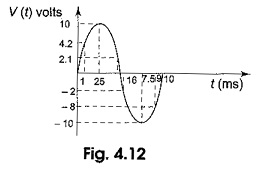In Fig. 4.12 during the positive cycle, the instantaneous values are positive and during the negative cycle, the instantaneous values are negative. In Fig. 4.12 shown at time 1 ms, the value is 4.2 V; the value is 10 V at 2.5 ms, – 2 V at 6 ms and – 10 V at 7.5 and so on.

### Peak Value:

The peak value of the sine wave is the maximum value of the wave during positive half cycle, or maximum value of wave during negative half cycle. Since the value of these two are equal in magnitude, a sine wave is characterized by a single peak value. The peak value of the sine wave is shown in Fig. 4.13; here the peak value of the sine wave is 4 V.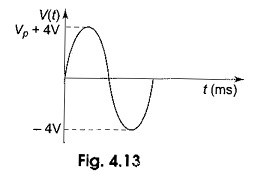### Peak to Peak Value:

The peak to peak value of a sine wave is the value from the positive to the negative peak as shown in Fig. 4.14. Here the peak to peak value is 8 V.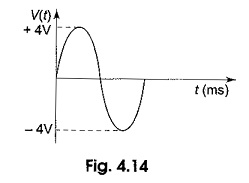### Average Value:

In general, the average value of any function υ(t), with period T is given by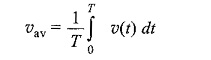That means that the average value of a curve in the X- Y plane is the total area under the complete curve divided by the distance of the curve. The average value of a sine wave over one complete cycle is always zero. So the average value of a sine wave is defined over a half-cycle, and not a full cycle period.

The average value of the sine wave is the total area under the half-cycle curve divided by the distance of the curve.

The average value of the sine wave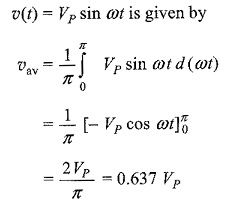The average value of a sine wave is shown by the dotted line in Fig. 4.15.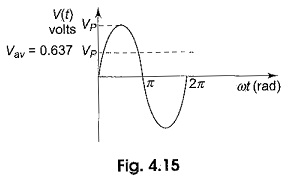### Root Mean Square Value or Effective Value:

The root mean square (rms) value of a sine wave is a measure of the heating effect of the wave. When a resistor is connected across a dc voltage source as shown in Fig. 4.17(a), a certain amount of heat is produced in the resistor in a given time. A similar resistor is connected across an ac voltage source for the same time as shown in Fig. 4.17(b). The value of the ac voltage is adjusted such that the same amount of heat is produced in the resistor as in the case of the dc source. This value is called the rms value.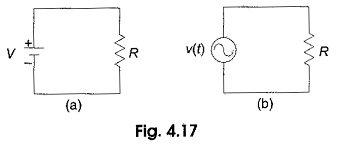That means the rms value of a sine wave is equal to the dc voltage that produces the same heating effect. In general, the rms value of any function with period T has an effective value given by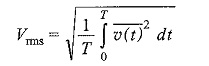Consider a function υ (t) = VP sin ωt

The rms value,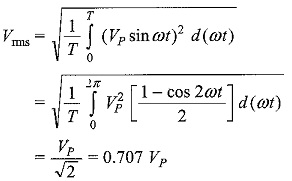If the function consists of a number of sinusoidal terms, that is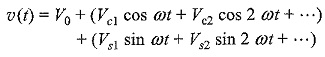The rms, or effective value is given by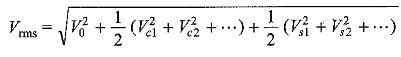### Peak Factor:

The peak factor of any waveform is defined as the ratio of the peak value of the wave to the rms value of the wave.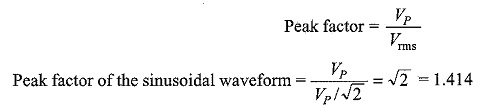### From Factor:

From factor of a waveform is defined as the ratio of rms value to the average value of  the wave.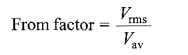From factor of a sinusoidal waveform can be found from the above relation.

For the sinusoidal wave,

Scroll to Top